## 10.5.1 Game Trees

In most literature, sequential games are formulated in terms of game trees. A state-space representation, which is more in alignment with the representations used in this chapter, will be presented in Section 10.5.2. The tree representation is commonly referred to as the extensive form of a game (as opposed to the normal form, which is the cost matrix representation used in Chapter 9). The representation is helpful for visualizing many issues in game theory. It is perhaps most helpful for visualizing information states; this aspect of game trees will be deferred until Section 11.7, after information spaces have been formally introduced. Here, game trees are presented for cases that are simple to describe without going deeply into information spaces.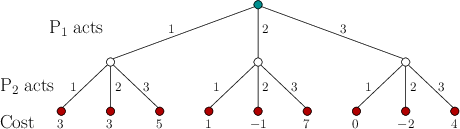Before a sequential game is introduced, consider representing a single-stage game in a tree form. Recall Example 9.14, which is a zero-sum,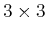matrix game. It can be represented as a game tree as shown in Figure 10.12. At the root,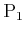has three choices. At the next level,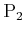has three choices. Based on the choices by both, one of nine possible leaves will be reached. At this point, a cost is obtained, which is written under the leaf. The entries of the cost matrix, (9.53), appear across the leaves of the tree. Every nonleaf vertex is called a decision vertex: One player must select an action.

There are two possible interpretations of the game depicted in Figure 10.12:

1. Before it makes its decision,knows which action was applied by. This does not correspond to the zero-sum game formulation introduced in Section 9.3 becauseseems as powerful as nature. In this case, it is not equivalent to the game in Example 9.14.
2.does not know the action applied by. This is equivalent to assuming that bothandmake their decisions at the same time, which is consistent with Formulation 9.7. The tree could have alternatively been represented withacting first.

Now imagine thatandplay a sequence of games. A sequential version of the zero-sum game from Section 9.3 will be defined by extending the game tree idea given so far to more levels. This will model the following sequential game:

Formulation 10..3 (Zero-Sum Sequential Game in Tree Form)
1. Two players,and, take turns playing a game. A stage as considered previously is now stretched into two substages, in which each player acts individually. It is usually assumed thatalways starts, followed by, thenagain, and so on. Player alternations continue until the game ends. The model reflects the rules of many popular games such as chess or poker. Let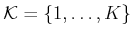denote the set of stages at whichandboth take a turn.
2. As each player takes a turn, it chooses from a nonempty, finite set of actions. The available set could depend on the decision vertex.
3. At the end of the game, a cost foris incurred based on the sequence of actions chosen by each player. The cost is interpreted as a reward for.
4. The amount of information that each player has when making its decision must be specified. This is usually expressed by indicating what portions of the action histories are known. For example, ifjust acted, doesknow its choice? Does it know what actionchose in some previous stage?

The game tree can now be described in detail. Figure 10.13 shows a particular example for two stages (hence,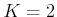and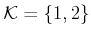). Every vertex corresponds to a point at which a decision needs to be made by one player. Each edge emanating from a vertex represents an action. The root of the tree indicates the beginning of the game, which usually means thatchooses an action. The leaves of the tree represent the end of the game, which are the points at which a cost is received. The cost is usually shown below each leaf. One final concern is to specify the information available to each player, just prior to its decision. Which actions among those previously applied by itself or other players are known?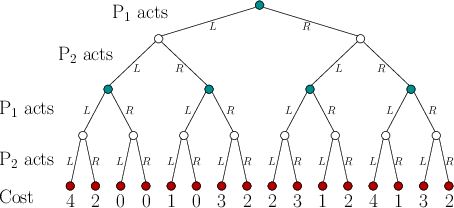For the game tree in Figure 10.13, there are two players and two stages. Therefore, there are four levels of decision vertices. The action sets for the players are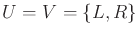, for left'' and right.'' Since there are always two actions, a binary tree is obtained. There are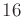possible outcomes, which correspond to all pairwise combinations of four possible two-stage plans for each player.

For a single-stage game, both deterministic and randomized strategies were defined to obtain saddle points. Recall from Section 9.3.3 that randomized strategies were needed to guarantee the existence of a saddle point. For a sequential game, these are extended to deterministic and randomized plans, respectively. In Section 10.1.3, a (deterministic) plan was defined as a mapping from the state space to an action space. This definition can be applied here for each player; however, we must determine what is a state'' for the game tree. This depends on the information that each player has available when it plays.

A general framework for representing information in game trees is covered in Section 11.7. Three simple kinds of information will be discussed here. In every case, each player knows its own actions that were applied in previous stages. The differences correspond to knowledge of actions applied by the other player. These define the state'' that is used to make the decisions in a plan.

The three information models considered here are as follows.

1. [] Alternating play: The players take turns playing, and all players know all actions that have been previously applied. This is the situation obtained, for example, in a game of chess. To define a plan, let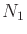and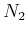denote the set of all vertices from whichandmust make a decision, respectively. In Figure 10.13,is the set of dark vertices andis the set of white vertices. Let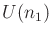and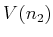be the action spaces forand, respectively, which depend on the vertex. A (deterministic) plan foris defined as a function,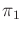, onthat yields an action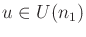for each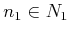. Similarly, a (deterministic) plan foris defined as a function,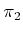, onthat yields an action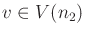for each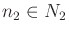. For the randomized case, let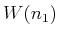and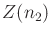denote the sets of all probability distributions overand, respectively. A randomized plan foris defined as a function that yields some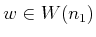for each. Likewise, a randomized plan foris defined as a function that maps frominto.

2. [] Stage-by-stage: Each player knows the actions applied by the other in all previous stages; however, there is no information about actions chosen by others in the current stage. This effectively means that both players act simultaneously in each stage. In this case, a deterministic or randomized plan foris defined as in the alternating play case; however, plans forare defined as functions on, instead of. This is because at the time it makes its decision,has available precisely the same information as. The action spaces formust conform to be dependent on elements of, instead of; otherwise,would not know what actions are available. Therefore, they are defined as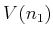for each.

3. [] Open loop: Each player has no knowledge of the previous actions of the other. They only know how many actions have been applied so far, which indicates the stage of the game. Plans are defined as functions on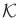, the set of stages, because the particular vertex is not known. Note that an open-loop plan is just a sequence of actions in the deterministic case (as in Section 2.3) and a sequence of probability distributions in the randomized case. Again, the action spaces must conform to the information. Thus, they are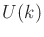and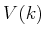for each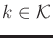.
For a single-stage game, as in Figure 10.12, the stage-by-stage and open-loop models are equivalent.

Subsections
Steven M LaValle 2020-08-14